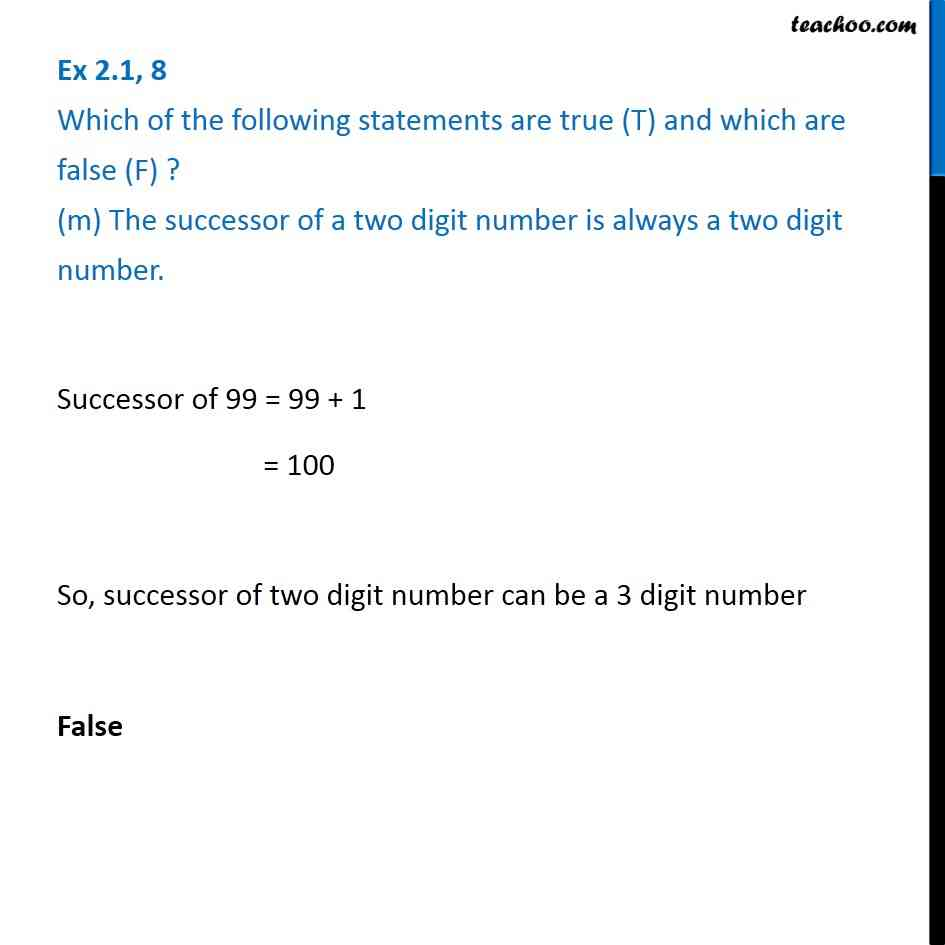1. Chapter 2 Class 6 Whole Numbers
2. Serial order wise
3. Ex 2.1

Transcript

Ex 2.1, 8 Which of the following statements are true (T) and which are false (F) ? (m) The successor of a two digit number is always a two digit number. Successor of 99 = 99 + 1 = 100 So, successor of two digit number can be a 3 digit number False

Ex 2.1

Chapter 2 Class 6 Whole Numbers
Serial order wise